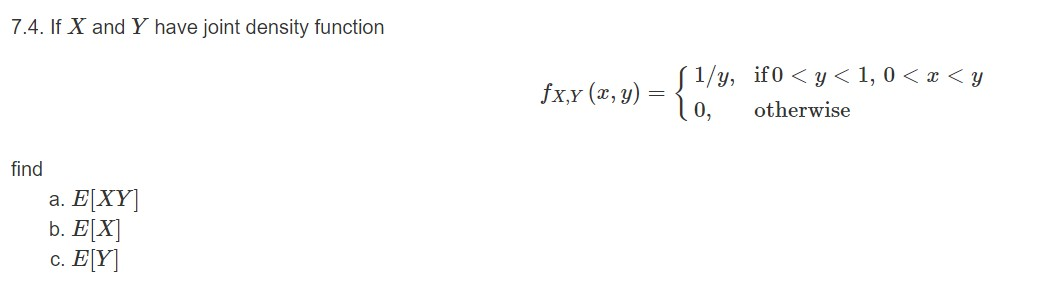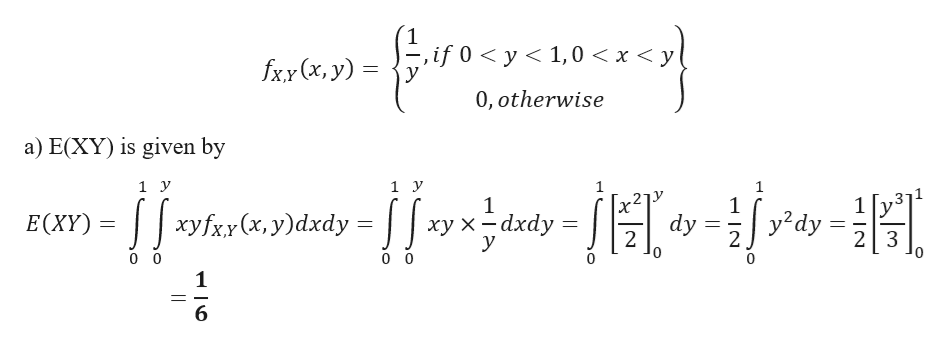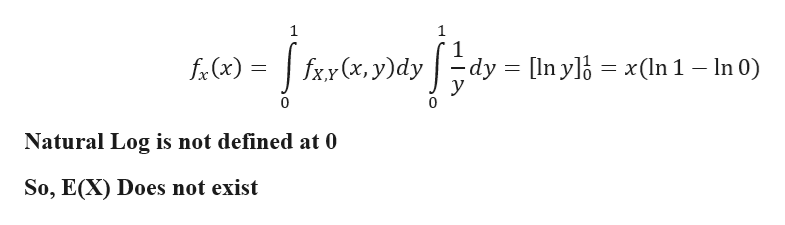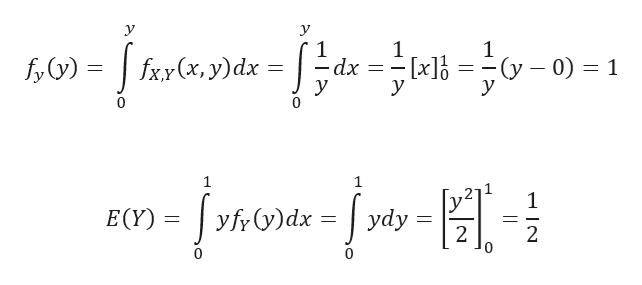# 7.4. If X and Y have joint density function( 1/y, if0 < y < 1, 0 < x < yo,fx,Y (x, y)otherwisefinda. E[XY]b. E[X]c. E[Y]

Question
8 views

3help_outlineImage Transcriptionclose7.4. If X and Y have joint density function ( 1/y, if0 < y < 1, 0 < x < y o, fx,Y (x, y) otherwise find a. E[XY] b. E[X] c. E[Y] fullscreen
check_circle

star
star
star
star
star
1 Rating
Step 1

Given Joint Density functionhelp_outlineImage Transcriptioncloseif 0 < y < 1,0 < x < y! fxy (x, y) = 0, otherwise a) E(XY) is given by - ||shotanar-||orjar-||-i{r»-| Sordy= E(XY) = || xyfxx (x, y)dxdy =: хух —dxdy dy fullscreen
Step 2

b) E(X) is given by

Computing Marginal density of xhelp_outlineImage Transcriptionclosef-(x) = | fxx(x, y)dy | fxx (x, y)dy dy = [In ylb = x(In 1 – In 0) Natural Log is not defined at 0 So, E(X) Does not exist fullscreen
Step 3

c)E(Y) is given by

Computing Ma...help_outlineImage Transcriptionclosey -(y – 0) = 1 -dx fxx(x, y)dx : y fy(y) = r0m- įmou-įso-| E(Y) = | yfrV)dx = | ydy 2 fullscreen

### Want to see the full answer?

See Solution

#### Want to see this answer and more?

Solutions are written by subject experts who are available 24/7. Questions are typically answered within 1 hour.*

See Solution
*Response times may vary by subject and question.
Tagged in

### Other